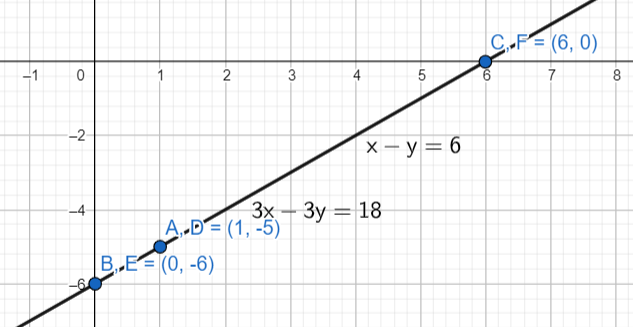QUESTION

# Solve the following pair of linear equations in two variables (by graph) :x – y = 6, 3x – 3y = 18

Hint: The given question is related to the representation of a linear equation on a cartesian plane. Represent the linear equations on the cartesian plane and find their point of intersection. The point of intersection of the two lines will be the solution of the pair of equations.

Before proceeding with the solution, let’s understand some important concepts of linear equations in two variables. A pair of linear equations in two variables represents a pair of straight lines on the cartesian plane. If the lines intersect at one point, then the pair of linear equations has exactly one solution. If the lines are parallel to each other, then the lines do not have any real solution. If the lines are coincident, then there are an infinite number of solutions.

Now, coming to the question, the pair of linear equations given to us is: x – y = 6 ………. (i)

and 3x – 3y = 18 ……… (ii).

Now, to draw the graph, we will find any three points lying on the lines and join them.

Consider the first equation: x – y = 6.

To find the first point, we will substitute x = 1 in equation (i) .

On substituting x = 1 in equation (i) , we get 1 – y = 6.

$\Rightarrow y=1-6$

$\Rightarrow y=-5$

So, A(1, -5) is the first point.

For the second point, we will substitute x = 0 in equation (i) .

On substituting x = 0 in equation (i) , we get 0 – y = 6.

$\Rightarrow y=-6$

So, B(0, -6) is the second point.

For the third point, we will substitute x = 6 in equation (i) .

On substituting x = 6 in equation (i) , we get 6 – y = 6.

$\Rightarrow y=0$

So, C(6, 0) is the third point.

Now, we will consider the second equation: 3x – 3y = 18.

To find the first point, we will substitute x = 1 in equation (ii) .

On substituting x = 1 in equation (ii) , we get 3 – 3y = 18.

$\Rightarrow 3y=3-18$

$\Rightarrow 3y=-15$

$\Rightarrow y=-5$

So, D(1, -5) is the first point.

For the second point, we will substitute x = 0 in equation (ii) .

On substituting x = 0 in equation (ii) , we get 0 – 3y = 18.

$\Rightarrow 3y=-18$

$\Rightarrow y=-6$

So, E(0, -6) is the second point.

For the third point, we will substitute x = 6 in equation (ii) .

On substituting x = 6 in equation (ii) , we get 18 – 3y = 18.

$\Rightarrow y=0$

So, F(6, 0) is the third point.

Now, we will plot these lines on a graph.From the diagram, we can clearly see that the lines represented by the pair of linear equations are coincident. Hence, there are infinite solutions to the given pair of linear equations in two variables.

Note: While choosing the points try to choose the points in such a way that the x- coordinates or the y – coordinates of the points in the two equations are the same. This helps in proper comparison of the lines.